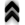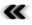## Chapter 7Dynamics in Action

He that will not apply new remedies must expect new evils.
Francis Bacon

All of the descriptions of dynamics given in Chapter 5 can be rendered using the simple concept of curves (Deﬁnition 45) associated with the evolution of the system, whether they appear merely as a means to track evolution or are seen as an essential component such as representatives of the ﬂow (Deﬁnition 67) driving evolution.

The following expositions will focus on the use of the Hamiltonian formalism, where curves, also called trajectories, are an essential component of modelling, since the whole phase space is subject to evolution due to the Hamiltonian ﬂow. Since here an individual curve, which is an integral curve (Deﬁnition 63) of the Hamiltonian vector ﬁeld (Deﬁnition 62), is a representative of the ﬂow, the evolution of any point along the curve is described by a simple shift along the curve. Since the integral curves of a Hamiltonian vector ﬁelds never intersect (with the exception of singularities), the evolution of every point is uniquely determined by knowing the integral curve.

Thus, using a discretization of the domain of interest as described in Section 6.5, it is possible to derive the evolution of a dynamic system in the environment of digital computers. However, the theoretical and practical considerations hampering direct utilization still apply.

Thus the reduction such as due to Boltzmann’s equation becomes a natural choice for further considerations. Boltzmann’s equation, however, also proved to be elusively diﬃcult to solve in a rigorous manner. This resulted in intense investigation of properties which could be extracted without actually solving the equation, or the gauging of the appropriateness of approximations.